Goubau TM0 Wire Wave

April 15, 2014

It is well known (Stratton 1941, Sommerfeld 1952) that an infinite straight cylindrical conductor embedded in a homogeneous dielectric medium supports an infinity of guided electromagnetic modes. Of these modes, only the circularly symmetric TM0 mode with propogation constant nearly equal to that of a plane wave in the external dielectric, or the principal TM mode, has low enough loss to be practically useful. While the loss of the principal mode is quite low, the fields extend radially outward to significant distances hindering the usefulness of this mode as a communications line as compared to say the coaxial line. However, Goubau (1950) demonstrated that the field extension of this mode can be reduced significantly by coating the cylindrical wire with a thin layer of dielectric. Goubau presents very useful graphical results for both the bare wire wave and coated wire cases with a function F(γ'ρ) defined to assist with calculation of fractional power extension outside the wire. Approximations possibly affecting the accuracy of some results were used for the coated wire case.

Fields

The radial field plots below show an example with f = 1GHz for a 1 mm radius copper wire comparing the coated wire case with an uncoated wire. The exact transcendental equations for both the bare wire and Goubau coated wire cases shown below were solved numerically for the propogation constant β with no approximations for either the bare wire or Goubau coated wire cases. The approximate expressions for β provided by Goubau were used as the starting value for numerical complex root searching with the transcendental equation for the coated wire. Accuracy of the results was checked against the transcendental equations. The numerical results based on the exact transcendental equation for the example are very close to the results obtained from the approximate Goubau expressions.

Field values shown are normalized to the total conduction current in the wire cross-section. While the Eρ(ρ) and Hφ(ρ) field values are comparable for the coated and uncoated cases near the wire up to radial distances of ~ 100mm and drop off as ~ 1/ρ for these closer radial distances, for greater distances these field components for the coated wire drop very quickly compared to the uncoated wire. In the final medium (ε=1) the ratio of Eρ/Hφ which is the longitudinal (axial) impedance is very nearly 376 Ω, the free space intrinsic impedance indicating that the field phasefronts outside the wire are very nearly normal to the wire.

The mode propogation loss (β_imaginary) is roughly twice as high for the coated case compared to the bare wire case but is about 16 times lower than that of coaxial line (ignoring any loss in the dielectric) with the same thickness dielectric but with outer shielding conductor: For the example above:
```
loss (bare wire wave):    0.012 dB/m
loss (coated wire wave):  0.021 dB/m
loss (coax):              0.33  dB/m
```Axial Power Charts

The charts below show the cumulative axial power as a function of radial distance for the same examples as above. Power calculations use the exact solutions for fields obtained above and exact integrals for total power. Both charts show the power values normalized to the total current amplitude squared (at an arbitrary z location) as well as the fraction of the total axial power flow contained in a circular area of radius ρ. The second chart for the coated conductor also shows the power flow in the coating layer Pzi, power in the external region Pze and total power Pztot as a function of radius and the fraction of the external power relative to the total power transmitted in the coating and external regions. It is obvious that the coating layer reduces the radial field extension considerably compared to the uncoated wire and this field extension is determined by the imaginary part of the transverse propogation constant λ3 in the external dielectric material (λ3 and β are determined by the solution of the transcendental equation .. see below). Goubau (1950) provides approximate design charts for general values of coating thickness and dielectric constant. The field and power calculations presented here use the exact transcendental equations for the propogation constant and exact field expressions for any radial distance ρ. Results agree closely with Goubau's graphs. For both cases, for smaller values of ρ the power in a radius ρ in the external dielectric medium has a ln(ρ/a) dependence where a is the radius at the interface to the external dielectric medium. The dependence in this region can be easily derived from Ampere's law with |I|^2 being the total current in the central conductor and is: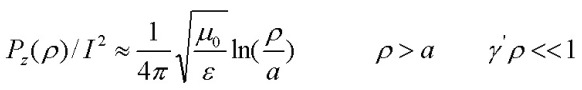Note that in the small ρ region, Pz(ρ) only depends on the geometry factor ρ/a and not the mode transverse propogation constant λ. However for large values of ρ for which γ'ρ ~ 1, the field dependence becomes exponential with radial distance and the radially integrated total axial power quickly approaches a finite limit. Simplifying the expression provided by Goubau (1950) for the lossless coated conductor case, the total power is: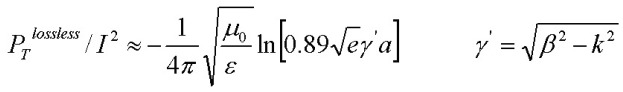In this lossless approximation for the coated wire case, the transverse propogation constant in the final dielectric medium is pure imaginary (λ3 = jγ'). As an estimate for the radial distance containing the full power, the small γ'ρ approximation Pz(ρ) can be equated to the total power value Pt which leads to: ργ' ~ 0.7. A calculator can be used to solve for the TM mode and calculate the power extension at any radial distance ρ.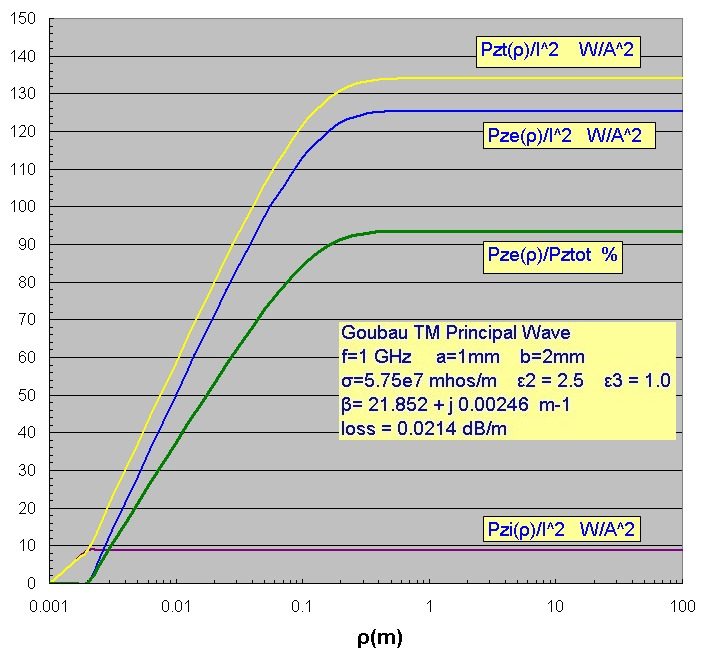Equations (for non-magnetic media)

With field components of the form:where β is the complex mode propogation constant, and bulk propogation constants given by: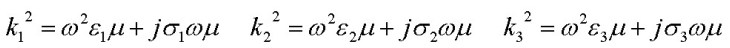The mode propogation constants for the axially symmetric TM modes for the bare wire are given by the solutions of:The mode propogation constants for the axially symmetric TM modes for the Goubau coated wire configuration are given by the solutions of: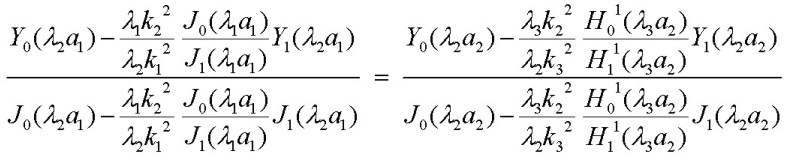where the transverse propogation constants are: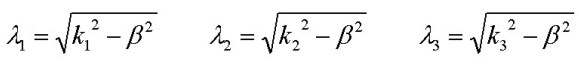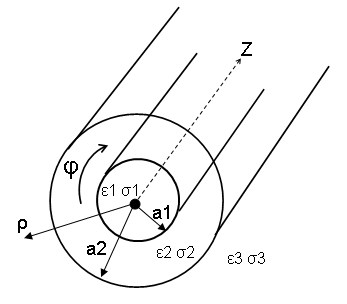Reference:
• Electromagnetic Theory, J. Stratton, 1941, McGraw Hill, pp. 524-536, 546-554
• Surface Waves and Their Application to Transmission Lines, G. Goubau, J. Appl. Phys, V21, 1950, p.1119
• Electrodynamics, A. Sommerfeld, Lectures on Theoretical Physics, 1952, VIII Academic Press, pp 177-185
• Field Theory of Guided Waves, R. E. Collin, 1991, IEEE Press, pp.697-700, 718-720